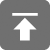# 工学1号馆

home

## C语言常量与指针

Wu Yudong    June 27, 2015     C   349

C语言功能强大而丰富，还表现在const与指针的结合使用上，对不同的问题，它提供不同的保护，特别有用的是指向常量的指针

### 指向常量的指针

int num = 5;
const int limit = 500;
int *pi;
const int *pci;
pi = &num;    //指向整数
pci = &limit; //指向整数常量

#include <stdio.h>
int main(void)
{
int num = 5;
const int limit = 500;
int *pi;
const int *pci;
pi = &num;    //指向整数
pci = &limit; //指向整数常量
printf("  num - Address:%p   value:%d\n", &num, num);
printf("limit - Address:%p   value:%d\n", &limit, limit);
printf("   pi - Address:%p   value:%p\n", &pi, pi);
printf("  pci - Address:%p   value:%p\n", &pci, pci);
return 0;
}

• pci可以被修改为指向不同的整数常量
• pci可以被修改为指向不同的非整数常量
• 可以解引pci以读取数据
• 不能解引pci从而修改它指向的数据

const int *pci = int const *pci

### 指向非常量的常量指针

int num;
int *const cpi = &num;

const int limit = 500;
int * const cpi = &limit;

### 指向常量的常量指针

const int * const cpci = &limit;

int num;
const int * const cpci = &num;

### 指向“指向常量的常量指针”的指针

#include <stdio.h>
int main(void)
{
const int limit = 500;
const int * const cpci = &limit;
const int * const * pcpci = &cpci;
printf("%d\n", *cpci);
printf("%d\n", **pcpci);
return 0;
}

 指针类型 指针是否可修改 指向指针的数据是否可修改 指向非常量的指针 是 是 指向常量的指针 是 否 指向非常量的常量指针 否 是 指向常量的常量指针 否 否

### 举例说明

#include<stdio.h>
#include<stdlib.h>
struct a
{
int x;
};
const struct a * function(void)
{
struct a *ptr;
if((ptr = (struct a *)malloc(sizeof(struct a))) == NULL)
exit(1);
ptr->x = 0;
return ptr;
}
int main(void)
{
int y;
const struct a *ptr;
ptr = function();
y = ptr->x;
return 0;
}

int main(void)
{
int y;
const struct a *ptr;
ptr = function();
ptr->x = 1;
return 0;
}

error: assignment of read-only location ‘*ptr’

int main(void)
{
int y;
struct a *ptr;
ptr = function();
ptr->x = 1;
return 0;
}

warning: assignment discards qualifiers from pointer target type

int main(void)
{
struct a *ptr;
ptr = (struct a *) function();
ptr->x = 1;
return 0;
}

#include<stdio.h>
#include<stdlib.h>
struct a
{
int x;
};
struct b
{
const struct a *nested_ptr;
};
const struct a * function(void)
{
struct a *ptr;
if((ptr = (struct a *) malloc(sizeof(struct a))) == NULL){
exit(1);
}
ptr->x = 0;
return ptr;
}
void do_something(const struct b *ptr)
{
const struct a *x = ptr->nested_ptr;
}
int main(void)
{
struct b b_obj;
b_obj.nested_ptr = function();
do_something(&b_obj);
return 0;
}

void do_something(const struct b * const ptr);

int main(void)
{
const struct a obj = [ 5 ];
return obj.x;
}

int main(void)
{
const struct a obj = [ 5 ];
const struct a *ptr_a = &obj;
const struct a *ptr_b = function();
return ptr_a->x;
}

const struct a * const function(void);

void fill_in(const struct a **location)
{
*location = function();
}
int main(void)
{
const struct a *ptr;
fill_in(&ptr);
return 0;
}

void fill_in(const struct a ** const location)
{
*location = function();
}

1、结构体是常量的，内容不能修改

2、指向该结构体的指针，通过location指向，*location不是常量，可以修改

3、变量location是常量的，意味着不能被修改

void fill_in(const struct a * const * const location)
{
*location = function();
}

error: assignment of read-only location ‘*location’ （由于*location也是常量，所以会得到gcc error）

void make_use_of(const struct a * const *location)
{
const struct a * const ptr_a = *location;
const struct a *ptr_b = *location;
ptr_b = NULL;
location = NULL;
}

1、结构体是常量的，内容不能修改

2、指向该结构体的指针，通过location指向，*location也是常量，不可以修改

3、变量location是非常量，意味着可以被修改

4、局部变量ptr_a是常量，不可以被修改

4、局部变量ptr_a不是常量，可以被修改

### 参考资料

《C和指针》